Journal DetailsFormat
Journal
eISSN
2444-8656
First Published
01 Jan 2016
Publication timeframe
2 times per year
Languages
EnglishOpen Access

# MHD 3-dimensional nanofluid flow induced by a power-law stretching sheet with thermal radiation, heat and mass fluxes

###### Accepted: 18 Dec 2019
Journal DetailsFormat
Journal
eISSN
2444-8656
First Published
01 Jan 2016
Publication timeframe
2 times per year
Languages
English

In this article, the three-dimensional Magnetohydrodynamics flow of a nanofluid over a horizontal non-linearly stretching sheet in bilateral directions under boundary layer approximation is addressed. A two-phase model has been used for the nanofluid. The influences of thermophoresis, Brownian motion and thermal radiation on heat and mass transfers are considered. Two different cases for the heat and mass transfers are studied. In the first case, uniform wall temperature and zero nanoparticles flux due to thermophoresis are considered. In the second case, prescribed heat and mass fluxes at the boundary are considered. By using the appropriate transformations, a system of non-linear partial differential equations along with the boundary conditions is transformed into coupled non-linear ordinary differential equations. Numerical solutions of the self-similar equations are obtained using a Runge–Kutta method with a shooting technique. Our results for special cases are compared with the available results in the literature, and the results are found to be in good agreement. It is observed that the pertaining parameters have significant effects on the characteristics of flow, heat and mass transfer. The results are presented and discussed in detail through illustrations.

#### MSC 2010

Introduction

Boundary layer flow over a stretching surface has various trade and technical applications, such as metal and polymer extrusion, paper production, and many others.

Also, it has important applications in engineering and manufacturing processes. Such processes are wire and fibre covering, foodstuff dispensation, heat-treated materials travelling between a feed roll and a wind-up roll or materials manufactured by extrusion, glass fibre production, cooling of metallic sheets or electronic chips, crystal growing, drawing of plastic sheets and so on. The quality of the final product with the desired features in these processes depends on the degree of freezing in the process and the process of stretching.

Flow past a linearly stretching sheet was first studied analytically by Crane (1970). Gupta and Gupta (1977) mentioned that in all realistic situations, the stretching mechanism is not linear. Later on, several investigators, Hamad and Pop (2010), Turkyilmazoglu (2013), Pal and Mandal (2015), Waqas et al. (2016), studied such type of flow problems either with heat or with mass transfer effects.

The main characteristic of nanofluid (a combination of nanoparticles having size 1–50 nm and liquid) is to improve the base fluid's thermal conductivity. Usually, ethylene glycol, oil, water, and so on are taken as base fluids that have limited heat transfer properties due to their low thermal conductivity. By adding nanoparticles into the base fluid, Masuda et al. (1993) first detected the change in viscosity and thermal conductivity of such fluids. The abnormal increase in the thermal conductivity occurs by the dispersion of nanoparticle, which was first observed by Choi and Eastman (1995). In case of nanofluids, Buongiorno (2006) studied the convective heat transfer and indicated that the Brownian motion, thermophoresis as the most important means for the unpredicted heat transfer augmentation. Later on, different aspects of nanofluid flow were addressed by several researchers. Considering heat and mass transfer, Das (2012) pointed out the slip effects in nanofluid flow. By the finite element method, Rana and Bhargava (2012) studied the nanofluid flow towards a non-linearly stretched surface. Nadeem and Lee (2012) studied the steady nanofluid flow over an exponentially stretched sheet. Considering the convective boundary condition (CBC), Mustafa et al. (2013) discussed nanofluid flow over an exponentially stretched surface. Using the Jeffrey's model for non-Newtonian fluid, Nadeem et al. (2014) investigated nanofluid flow over a stretching surface to obtain a numerical solution. Magnetohydrodynamics (MHD) plays a great role in several areas particularly in geophysics, medicine, engineering and many others [Hayat et al. (2016)]. Magneto nanofluids have significant applications in drug delivery, cancer therapy, enhancement of magnetic resonance and magnetic cell separation (Hayat et al. 2016). In 2013, Khan et al. analysed the effects of a magnetic field, applied externally on nanofluid flow due to a stretching sheet considering a non-similar solution of the problem. Influences of the magnetic field on Cu-water flow were analysed by Sheikholeslami et al. (2014). Using the Buongiorno's model, in 2016, Sheikholeslami and his co-authors described the unsteady flow of nanofluid in the presence of a magnetic field. Ma et al. (2019) studied the consequences of the magnetic field on forced convection Ag-MgO nanofluid flow in a channel with heat transfer. Many other research papers on nanofluid with magnetic field effects can be found in the studies conducted by Ma et al. (2019) and Bhatti et al. (2018).

Thermal radiation affects the heat transfer characteristics significantly particularly in control of heat transfer, space technology and high-temperature processes (Das et al. 2014). Hayat et al. (2016) reported the combined effects due to Joule heating and solar emission on MHD thixotropic nanofluid flow. Radiative flows in companies with the external magnetic fields have wider applications in nuclear engineering, power technology, astrophysics, power generation and so on (Hayat et al. 2016).

The investigations described above are two-dimensional. Three-dimensional problem being more realistic and has already drawn the attention of several researchers. Wang (1984) first addressed the three-dimensional flow due to a bidirectional stretching sheet. Ariel (2007) obtained the solution for a three-dimensional flow caused by a stretching surface using a perturbation technique. Liu and Andersson (2008) analysed the heat transport of a 3-D flow due to a stretching surface. Ahamad and Nazar (2011) discussed the MHD 3-D flow of a viscoelastic fluid due to a stretching surface. In 2013, Hayat and his co-authors considered 3-D Oldroyd-B fluid flow past a stretching surface with a CBC. Considering the exponential temperature distribution, 3-D flow and heat transfer of a viscous fluid were investigated by Liu et al. (2013). Nadeem et al. (2013) analysed the 3-D Casson fluid flow due to a linearly stretching sheet in the presence of a magnetic field. Khan et al. (2014) obtained analytical and numerical solutions for a three-dimensional flow caused by a non-linearly stretching sheet. Khan et al. (2015) investigated three-dimensional flow of a nanofluid past a non-linearly stretching sheet and highlighted its applications to the solar energy industry. Existence of two solutions was reported by Raju et al. (2016) for flow due to a non-linearly stretching sheet. Considering thermal radiation and heat generation/absorption, Shehzad et al. (2016) analysed 3-D MHD flow of a Jeffrey nanofluid over a bidirectional stretching sheet.

The combined effects of MHD and thermal radiation on three-dimensional non-linear convective Maxwell nanofluid flow owing to a stretching sheet were reported by Hayat et al. (2017). The literature review reveals the fact that there is not so much work has been done on three-dimensional nanofluid flow caused by a non-linearly stretching sheet.

Motivated by this, in this article, we investigate the radiative heat transport of the MHD flow of a nanofluid past a stretching sheet with power-law velocity in two directions. The mathematical representations of the nanofluid model proposed by Buongiorno (2006) reflecting the contribution of nanoparticle volume fraction were used. This model also includes the effects of thermophoresis and the Brownian motion. The main objective here is to analyse the heat transport features of the flow of a nanofluid owing to the collective influences of magnetic field and radiation. Two different cases are considered: In Case I, uniform surface temperature is considered. Due to thermophoresis, a flux of nanoparticles volume fraction at the sheet is assumed to be zero. This assumption controls the volume fraction of nanoparticles submissively rather than actively (Mansur and Ishak 2013; Nield and Kuznetsov 2014); on the other hand, in Case II, heat and mass fluxes are considered at the sheet. Suitable similarity transformations are considered to transform the highly non-linear partial differential equations into ordinary ones. Numerical results are obtained by a Runge–Kutta method with a shooting technique. For a clear understanding of the flow and heat transport characteristics, the obtained results are analysed in detail with their physical interpretation through Tables and Figures. It is observed that the physical parameters affect the heat and mass transport significantly.

Mathematical modelling

Let us consider a 3-D steady boundary layer nanofluid flow past a non-linearly stretching surface. In a usual notation, the governing equations of the problem can be written as $∂u∂x+∂υ∂y+∂w∂z=0$ \frac{{\partial u}}{{\partial x}} + \frac{{\partial \upsilon }}{{\partial y}} + \frac{{\partial w}}{{\partial z}} = 0 $u∂u∂x+υ∂u∂y+w∂u∂z=ν∂2u∂z2-σB02ρfu$ u\frac{{\partial u}}{{\partial x}} + \upsilon \frac{{\partial u}}{{\partial y}} + w\frac{{\partial u}}{{\partial z}} = \nu \frac{{{\partial ^2}u}}{{\partial{z^2}}} - \frac{{\sigma B_0^2}}{{{\rho _f}}}u $u∂υ∂x+υ∂υ∂y+w∂υ∂z=ν∂2υ∂z2-σB02ρfυ$ u\frac{{\partial \upsilon }}{{\partial x}} + \upsilon \frac{{\partial \upsilon}}{{\partial y}} + w\frac{{\partial \upsilon }}{{\partial z}} = \nu\frac{{{\partial ^2}\upsilon }}{{\partial {z^2}}} - \frac{{\sigma B_0^2}}{{{\rho_f}}}\upsilon $u∂T∂x+υ∂T∂y+w∂T∂z=α∂2T∂z2-1(ρc)f∂qr∂z+(ρc)p(ρc)f[DB∂C∂z∂T∂z+DTT∞(∂T∂z)2]$ u\frac{{\partial T}}{{\partial x}} + \upsilon \frac{{\partial T}}{{\partial y}}+ w\frac{{\partial T}}{{\partial z}} = \alpha \frac{{{\partial ^2}T}}{{\partial{z^2}}} - \frac{1}{{{{\left( {\rho c} \right)}_f}}}\frac{{\partial{q_r}}}{{\partial z}} + \frac{{{{(\rho c)}_p}}}{{{{(\rho c)}_f}}}\left[{{D_B}\frac{{\partial C}}{{\partial z}}\frac{{\partial T}}{{\partial z}} +\frac{{{D_T}}}{{{T_\infty }}}{{\left( {\frac{{\partial T}}{{\partial z}}}\right)}^2}} \right] $u∂C∂x+υ∂C∂y+w∂C∂z=DB∂2C∂z2+DTT∞∂2T∂z2$ u\frac{{\partial C}}{{\partial x}} + \upsilon \frac{{\partial C}}{{\partial y}}+ w\frac{{\partial C}}{{\partial z}} = {D_B}\frac{{{\partial ^2}C}}{{\partial{z^2}}} + \frac{{{D_T}}}{{{T_\infty }}}\frac{{{\partial ^2}T}}{{\partial {z^2}}} where the components of velocity in the x,y and z directions are u, υ and w, respectively; kinematic viscosity is ν, electrical conductivity is σ, ρf is the density, T denotes the temperature of the fluid, α denotes the thermal diffusivity, B0 = a/(x + y)1−n represents the variable magnetic field, the effectual heat capability of the nanoparticles is (ρc)p, and (ρc)f is the effectual heat capability of nanofluid; DB, DT are the diffusion coefficients due to the Brownian motion and the thermophoresis, respectively; qr represents the radiative heat flux, C is the nanoparticle volume fraction and T is the steady temperature of the inviscid free stream.

Using the Rosseland approximation, the expression for radiative heat flux can be written as $qr=-4σs3ke∂T4∂z$ {q_r} = - \frac{{4{\sigma _s}}}{{3{k_e}}}\frac{{\partial {T^4}}}{{\partial z}} where σs and ke are the Stefan–Boltzmann constant and the mean absorption coefficient, respectively. This investigation is restricted to the optically bulky fluid. For sufficiently small temperature difference linearisation of Eq. (6) can be performed. Using the Taylor's series expansion for T4 about T and ignoring the higher-order terms, we get $T4=4T∞3T-3T∞4.$ {T^4} = 4T_\infty ^3T - 3T_\infty ^4.

A. Boundary conditions for fluid flow

The boundary conditions can be written as $at z=0, u=uw=a(x+y)n,υ=υw=b(x+y)n,w=0 and as z→∞,u→0,υ→0$ {\rm{at\;\; }}z = 0,\,\,u = {u_w} = a{\left( {x + y} \right)^n},\upsilon ={\upsilon _w} = b{\left( {x + y} \right)^n},w = 0\,{\rm{ \;\;and}}\,\,{\rm{as\;\;}}z \to\infty ,u \to 0,\upsilon \to 0 uw and υw denote the velocities of stretching along the x and y directions, respectively, and n is a non-linearly stretching parameter and C is the uniform volume fraction for the nanoparticles in the inviscid free stream.

B. Boundary conditions for heat, mass transport

There are different types of heating process that specify the temperature distribution on the surface and ambient fluid. These are constant or prescribed wall temperature (CWT or PWT); constant or prescribed surface heat flux (CHF or PHF), CBC and Newtonian heating. In this problem, we have considered two cases of temperature distributions: CWT and CHF separately. Moreover, different methods are available for the active or passive control of nanoparticles, namely, constant or prescribed concentration at the wall (CHF or PWC); zero mass or normal flux of nanoparticles at the surface (ZMF); constant or prescribed mass flux (CMF or PMF). In this problem, two different boundary conditions, ZMF and CMF for mass transfer, are considered.

Therefore, the conditions at the boundary for temperature, volume fraction for nanoparticles are

Case I: $T=Tw, DB∂C∂z+DTT∞∂T∂z=0 at z=0 (CWT,ZMF) & T→T∞, C→C∞ as z→∞$ T = {T_w},\,{D_B}\frac{{\partial C}}{{\partial z}} + \frac{{{D_T}}}{{{T_\infty}}}\frac{{\partial T}}{{\partial z}} = 0\,{\rm{at}}\,\,z = 0\text{ (CWT, ZMF) & }T\to {T_\infty },\,C \to {C_\infty }\,\,{\rm{as}}\,\,z \to \infty

Case II: $∂T∂z=-Twα, ∂C∂z=-CwDB at z=0 (CHF,CMF) & T→T∞, C→C∞ as z→∞$ \frac{{\partial T}}{{\partial z}} = - \frac{{{T_w}}}{\alpha},\,\,\frac{{\partial C}}{{\partial z}} = -\frac{{{C_w}}}{{{D_B}}}\,\,{\rm{at}}\,\,z = 0\text{ (CHF, CMF) & }T \to {T_\infty},\,C \to {C_\infty }\,\,{\rm{as}}\,\,z \to \infty

where Tw and Cw are the temperature and concentration at the surface, respectively.

Let us define the similarity variable and similarity transformations as $η=aν(x+y)n-12z,$ \eta = \sqrt {\frac{a}{\nu }} {\left( {x + y} \right)^{\frac{{n - 1}}{2}}}z, $u=a(x+y)nf′(η), υ=a(x+y)ng′(η), w=-aν(x+y)n-12[n+12(f+g)+n-12η(f′+g′)],$ u = a{\left( {x + y} \right)^n}f'(\eta ),\,\upsilon = a{\left( {x + y}\right)^n}g'(\eta ),\,w = - \sqrt {a\nu } {\left( {x + y} \right)^{\frac{{n -1}}{2}}}\left[ {\frac{{n + 1}}{2}\left( {f + g} \right) + \frac{{n - 1}}{2}\eta\left( {f' + g'} \right)} \right], $θ(η)=T-T∞Tw-T∞$ \theta (\eta ) = \frac{{T - {T_\infty }}}{{{T_w} - {T_\infty }}} , $φ=C-C∞C∞$ \varphi = \frac{{C - {C_\infty }}}{{{C_\infty }}} (for Case-I) $T=T∞+Twα(νa(n+1)(x+y)n-1)12θ(η), C=C∞+CwDB(νa(n+1)(x+y)n-1)12φ(η)$ T = {T_\infty } + \frac{{{T_w}}}{\alpha }{\left( {\frac{\nu }{{a(n + 1){{(x +y)}^{n - 1}}}}} \right)^{\frac{1}{2}}}\theta (\eta ),\,C = {C_\infty } +\frac{{{C_w}}}{{{D_B}}}{\left( {\frac{\nu }{{a(n + 1){{(x + y)}^{n - 1}}}}}\right)^{\frac{1}{2}}}\varphi (\eta ) (for Case-II).

Using these transformations in Eqs (1)–(5) and also using the relation given by Eq. (6), we get the following self-similar equations $f‴+n+12(f+g)f″-n(f′+g′)f′-Mf′=0$ f''' + \frac{{n + 1}}{2}(f + g)f'' - n(f' + g')f' - {\rm{M}}f' = 0 $g‴+n+12(f+g)g″-n(f′+g′)g′-Mg′=0$ g''' + \frac{{n + 1}}{2}(f + g)g'' - n(f' + g')g' - {\rm{M}}g' = 0 $1Pr(1+43R)θ″+n+12(f+g)θ′+Nbθ′ϕ′+Ntθ′2=0$ \frac{1}{{\Pr }}(1 + \frac{4}{3}R)\theta '' + \frac{{n + 1}}{2}(f + g)\theta ' +{N_b}\theta '\phi ' + {N_t}{\theta '^2} = 0 $ϕ″+(n+1)2Sc(f+g)ϕ′+NtNbθ″=0.$ \phi '' + \frac{{(n + 1)}}{2}Sc(f + g)\phi ' + \frac{{{N_t}}}{{{N_b}}}\theta ''= 0. Here, the magnetic parameter is $M=σB02ρfa$ {\rm{M}} = \frac{{\sigma B_0^2}}{{{\rho_f}a}} , Prandtl number is $Pr=να$ \Pr = \frac{\nu }{\alpha } , $Nb=(ρc)pDBC∞(ρc)fν$ {N_b} = \frac{{{{({\rho _c})}_p}{D_B}{C_\infty }}}{{{{({\rho _c})}_f}\nu }} represents the Brownian motion parameter and $Nt=(ρc)pDT(Tw-T∞)(ρc)fvT∞$ {N_t} = \frac{{{{({\rho _c})}_p}{D_T}({T_w} - {T_\infty })}}{{{{({\rho _c})}_f}v{T_\infty }}} represents the thermophoresis parameter, radiation parameter is $R=4σsT∞3ke(ρc)fα$ {\rm{R}} = \frac{{4{\sigma _s}T_\infty^3}}{{{k_e}{{(\rho c)}_f}\alpha }} , $Sc=νDB$ Sc = \frac{\nu }{{{D_B}}} is the Schmidt number, $λ=ba$ \lambda = \frac{b}{a} denotes the ratio of rate of stretching along y-direction to that of x-direction.

The boundary condition becomes $f(η)=0, f′(η)=1 at η=0 and f′(η)→1 as η→∞$ f(\eta ) = 0,\,f'(\eta ) = 1\,{\rm{at}}\,\eta = 0\,{\rm{and}}\,f'(\eta ) \to1\,{\rm{as}}\,\eta \to \infty $g(η)=0, g′(η)=λ at η=0 and g′(η)→1 as η→∞$ g(\eta ) = 0,\,\,g'(\eta ) = \lambda \,\,{\rm{at}}\,\,\eta =0\,\,{\rm{and}}\,\,\,g'(\eta ) \to 1\,\,{\rm{as}}\,\,\eta \to \infty $θ(η)=1 and Nbϕ′(η)+Ntθ′(η)=0 at η=0 (for Case-I)$ \theta (\eta ) = 1\,{\rm{and }}{{\rm{N}}_{\rm{b}}}\phi '(\eta ) +{{\rm{N}}_{\rm{t}}}\theta '(\eta ) = 0\,{\rm{\;at\;}}\,\eta = 0\,{ (\text{for}\,\, \text{Case-I})} $θ′(η)=-1,ϕ′(η)=-1 at η=0 (for Case-II)$ \theta'(\eta) = -1, \phi'(\eta) = -1\; at \; \eta =0\;\;\;\;\mathrm{(for\;\; Case-II) } $θ(η)→0,ϕ(η)→0 as η→∞.$ \theta (\eta) \to 0 , \phi(\eta) \to 0 \;\; as \;\; \eta \to \infty. Dimensionless wall shear stresses, heat and mass fluxes are $Cfx=τzxρfuw2, Cfy=τzyρfνw2, Nu=(x+y)qwk(Tw-T∞), Sh=(x+y)jwDB(Cw-C∞)$ Cf_x = \frac{\tau_{zx}}{\rho_f u_w^2}, \; Cf_y = \frac{\tau_{zy}}{\rho_f \nu_w^2} , \; Nu = \frac{(x+y)q_w}{k(T_w -T_\infty)} , \; Sh = \frac{(x+y)j_w}{D_B(C_w - C_\infty)} where τzx and τzy are shear stresses at the wall and qw represents the heat flux at the wall, jw denotes the mass flux which are described as $τzx=μ(∂u∂z+∂w∂x)z=0,τzy=μ(∂ν∂z+∂w∂y)z=0,qw=-k(∂T∂y)z=0,jw=-DB(∂C∂y)z=0.$ \tau_{zx} = \mu \left( \frac{\partial u}{\partial z} + \frac{\partial w}{\partial x}\right)_{z=0},\tau_{zy} = \mu \left(\frac{\partial \nu}{\partial z} + \frac{\partial w}{\partial y} \right)_{z=0},q_w =-k \left( \frac{\partial T}{\partial y} \right)_{z=0},j_w = -D_B \left( \frac{\partial C}{\partial y}\right)_{z=0}. Finally, we get $CfxRex1/2=f″(0), CfyRey1/2λ3/2=g″(0), NuRex-1/2=-θ′(0), ShRex-1/2=-ϕ′(0)$ Cf_x Re_x^{1/2} =f '' (0), \; Cf_y Re_y^{1/2} \lambda^{3/2} = g'' (0), \; NuRe_x^{-1/2} = - \theta'(0), \; ShRe_x^{-1/2} = - \phi'(0) where the Reynolds numbers (local) along the directions of x,y are $Rex=uw(x+y)vf$ Re_x = \frac{u_w(x+y)}{v_f} and $Rey=uw(x+y)vf$ Re_y = \frac{u_w(x+y)}{v_f} , respectively. The far field component of velocity may be written in the form $w(x,t,∞)=-avy(x+y)n-1n+12[f(∞)+g(∞)]$ w(x,t,\infty) = - \sqrt{a v_y (x+y)^{n-1}} \frac{n+1}{2} [f(\infty) + g(\infty)]

The differential Eqs (11)–(14) are coupled and non-linear. Equations (11)–(14), together with the conditions (15a–15d), form a two-point boundary value problem. By converting it into an initial value problem, those equations are numerically solved using the Runge–Kutta method and the shooting technique by choosing an appropriate value for n.

Special cases

For λ = 0, the aforementioned equations represent the case of a 2-D flow.

For λ = 1, the system leads to the axisymmetric nanofluid flow owing to a non-linearly stretched surface.

Results and interpretations
Testing of numerical results

To test the validity of our numerical results, an assessment is made with the available results from the open literature for some special cases and presented in Table 1. Table 1 shows a complete agreement of our results with those of Khan et al. (2015) and Raju et al. (2016) in the absence of the magnetic field and thermal radiation.

Comparisons for the values of f″(0) and g″(0) in the absence of magnetic field

N λ f″(0) g″(0)
Khan et al. (2015) Raju et al. (2016) Present study Khan et al. (2015) Raju et al. (2016) Present study
1 0 −1 −1 −1 0 0 0
0.5 −1.22474 −1.2247431 −1.2247429 −0.612372 −0.6123721 −0.6123720
1.0 −1.414214 −1.4142141 −1.4142140 −1.414214 −1.4142140 −1.4142140
3 0 −1.624356 −1.6243560 −1.6243560 0 0 0
0.5 −1.989422 −1.9894221 −1.9894220 −0.994711 −0.9947110 −0.9947110
1.0 −2.297186 −2.2971860 −2.2971860 −2.297186 −2.2971860 −2.2971860

Grid independence test has been performed and presented through Table 2, which shows that the values of f″(0), g″(0) are the same for two different grid sizes (0.01, 0.03).

Comparisons of the values of f″(0) and g″(0) in the absence of the magnetic field for different grid sizes

N f″(0) g″(0)
Grid size 0.01 Grid size 0.03 Grid size 0.01 Grid size 0.03
1 0 −1 −1 0 0
0.5 −1.2247429 −1.2247429 −0.6123720 −0.6123720
1.0 −1.4142140 −1.4142140 −1.4142140 −1.4142140
3 0 −1.6243560 −1.6243560 0 0
0.5 −1.9894220 −1.9894220 −0.9947110 −0.9947110
1.0 −2.2971860 −2.2971860 −2.2971860 −2.2971860
Analysis of the results

To obtain in-depth features, the numerical results are presented graphically in Figures 2–12.

Figures 2(a–f) depict the effects of magnetic field on velocity components along with the directions of x and y axes, temperature and concentration fields. Linearly (n = 1) and non-linearly stretching (n = 3) cases are considered. As the Lorentz force (which resists the movement of fluid) increases (increase in magnetic parameter M), the velocity in both directions decreases [Figures 2(a,b)]. This result is similar to that of Mahanthesh et al. (2016). But with an increase in the magnetic field, an increase in temperature in the cases of CWT [Figure 2(c)] and PHF [Figure 2(d)] and concentration in the cases of ZMF [Figure 2(e)] and PMF [Figure 2(f)] is observed. The effect of the magnetic field on the temperature field is more pronounced in the PHF case. Owing to the occurrence of an externally applied magnetic field resistance to the stream is created, and as a result, it transformed some energy to heat energy. Consequently, the temperature increased with an increase in M [Figures 2(c,d)]. Concentration increases in the vicinity of the surface and rises to a maximum before it starts to decay and finally vanishes due to the thermophoresis effect [Figure 2(e)] in the case of ZMF. The nanoparticle volume fraction at the wall is negative, which can be verified by the boundary condition $φ′(0)=-NtNbθ′(0)$ \varphi '(0) = - \frac{{{N_t}}}{{{N_b}}}\theta '(0) . This is quite obvious, and similar results can be found in Kuznetsov and Nield (2014), and Mustafa et al. (2015). From these figures, it is also noticed that an increase in the parameter n (power-law index), velocities in both directions, temperature and concentration decrease.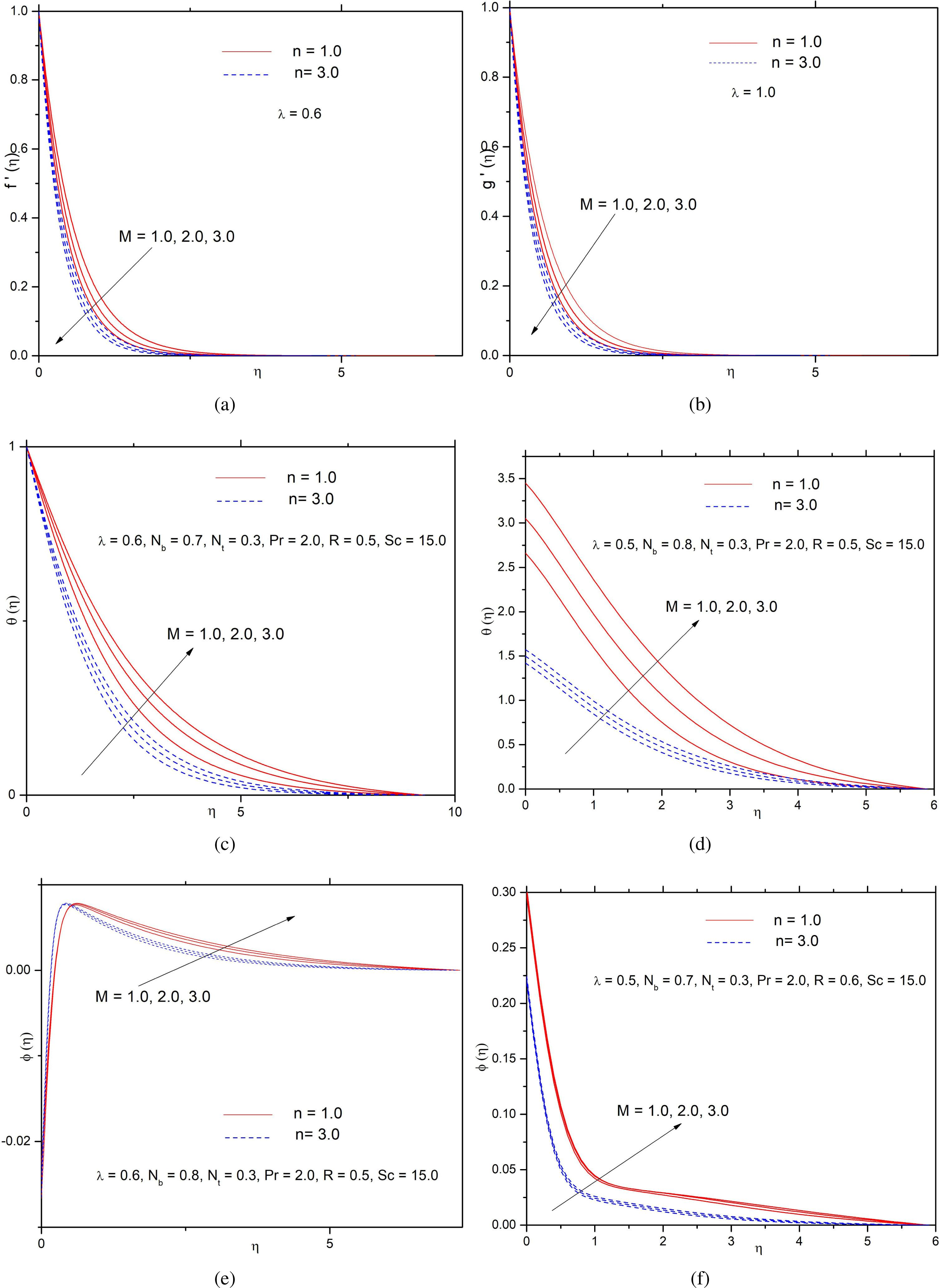Influences of magnetic parameter M on (a) f′(η), velocity in the direction of x (b) g′(η), velocity in the direction of y, temperature θ (η) for (c) CWT (d) PHF and nanoparticle volume fraction ϕ(η) for (e) ZMF and (f) PMF.

From Figures 3(a–f), it is clear that the fluid velocity in the direction of x-axis decreases [Figure 3(a)]; but it increases significantly in the y-directions [Figure 3(b)] owing to augment in the parameter λ. That is, an increase in the stretching ratio parameter λ helps to accelerate the flow in the vertical direction [Khan et al. (2015)]. Owing to the augmentation of stretching in the y-direction, pressure on flow increases, and hence, the temperature for both CWT [Figure 3(c)] and PHF [Figure 3(d)] cases and concentration for both ZMF [Figure 3(e)] and PMF [Figure 3(f)] cases decrease. The effect of stretching ratio parameter λ for both linearly (n = 1) and non-linearly (n = 3) stretching cases are exhibited through the figures. A significant effect in the thermal field is noted in the PHF case compared to that of the CWT case. The mixing tendency of cold fluid with the ambient fluid along the stretched surface increases with a rise in λ which causes to diminish the hotness of the liquid. The influence of λ on nanoparticle volume fraction is more pronounced in the PMF case compared to that of the ZMF case. Less penetration of nanoparticle is noted due to the augment in as the speedy flow in a downward direction brushes away the nanoparticles on the way to the surface.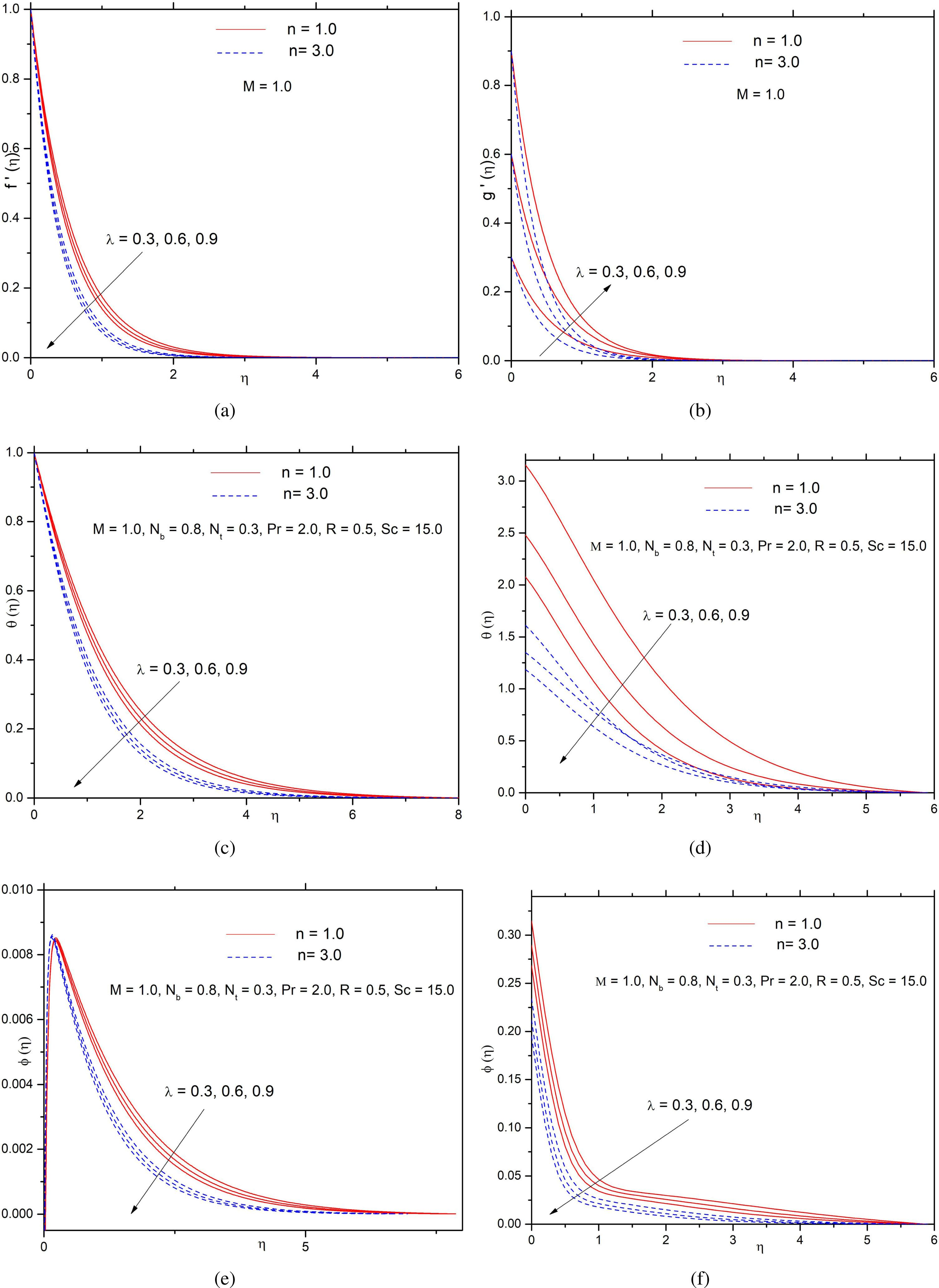Influences of λ, stretching ratio parameter on (a) f′(η), velocity in the direction of x (b) g′(η), velocity in the direction of y, temperature θ (η) for (c) CWT and (d) PHF and nanoparticle volume fraction ϕ(η) for (e) ZMF and (f) PMF.

The effect of n on velocity, temperature and concentration of the nanoparticles can be seen in Figures 4(a–d). Velocities along the directions of x [Figure 4(a)] and y [Figure 4(b)] are found to diminish with increasing n. The observation is similar to that of Khan et al. (2015) and Mahanthesh et al. (2016). As the power-law index n increases, larger shear force is experienced. As a result, skin friction coefficients in both the directions are augmented due to an increase in n. Temperature for the PHF case [Figure 4(c)] and nanoparticle volume fraction for PMF case [Figure 4(d)] also reduce by a rise in n.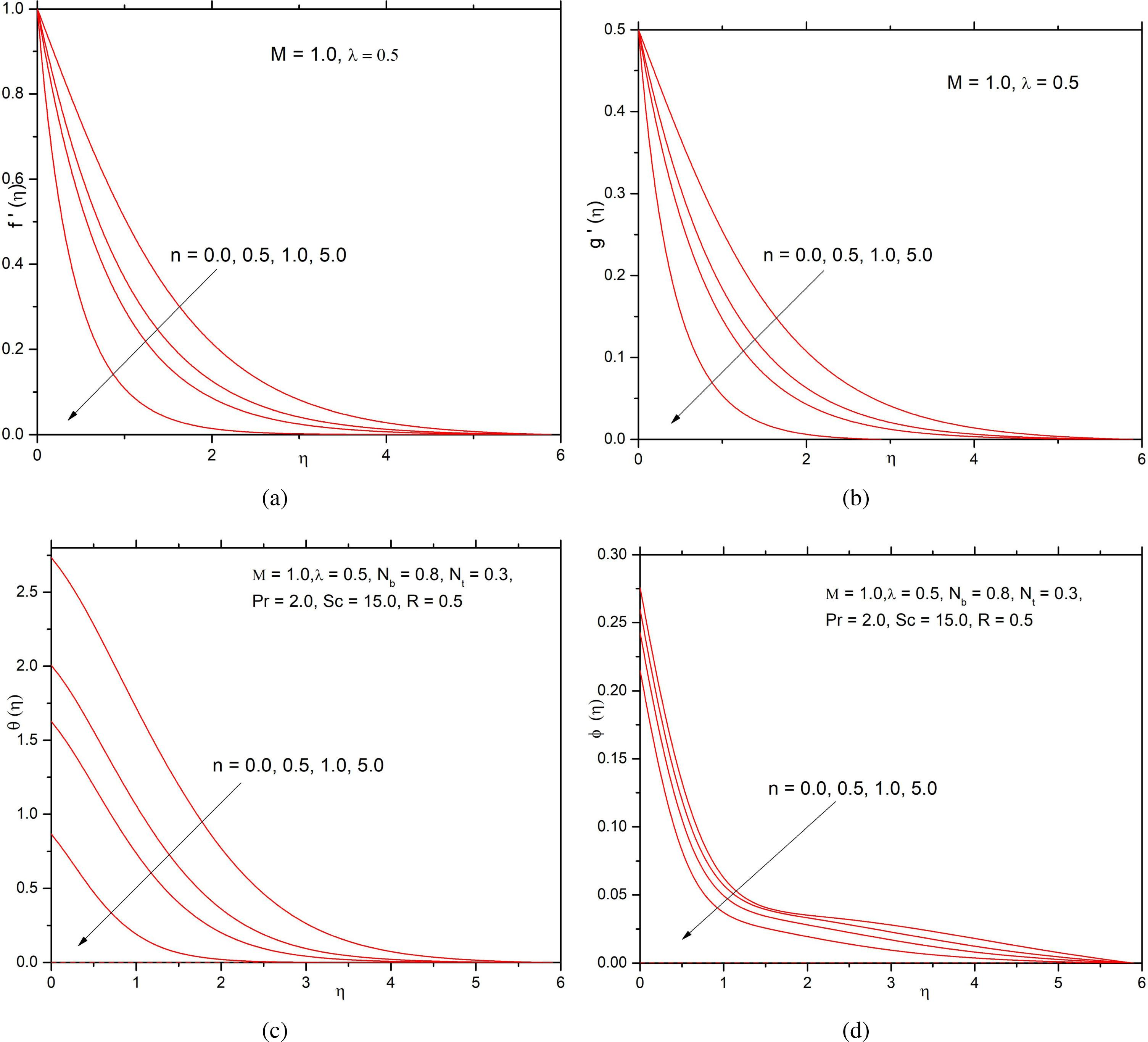Influences of n, non-linearly stretching parameter on (a) f′(η), velocity in the direction of x (b) g′(η), velocity in the direction of y, (c) θ (η), temperature for PHF and (d) nanoparticle volume fraction ϕ(η) for PMF.

From Figures 5(a–d), it is seen that the temperature [Figures 5(a,b)] and nanoparticle volume fraction [Figures 5(c,d)] are increasing functions of thermophoresis parameter Nt: The force responsible for the diffusion of nanoparticle owing to temperature ramp is recognised. Growth in the width of the boundary layer related to the temperature field is observed for higher values of the thermophoresis parameter Nt [Figures 5(a,b)]. Through a rise in Nt,, the thermal conductivity of the nanofluid increases due to the company of nanoparticles. As a result, the concentration of the fluid increases through the rise in Nt [Figures. 5(c,d)].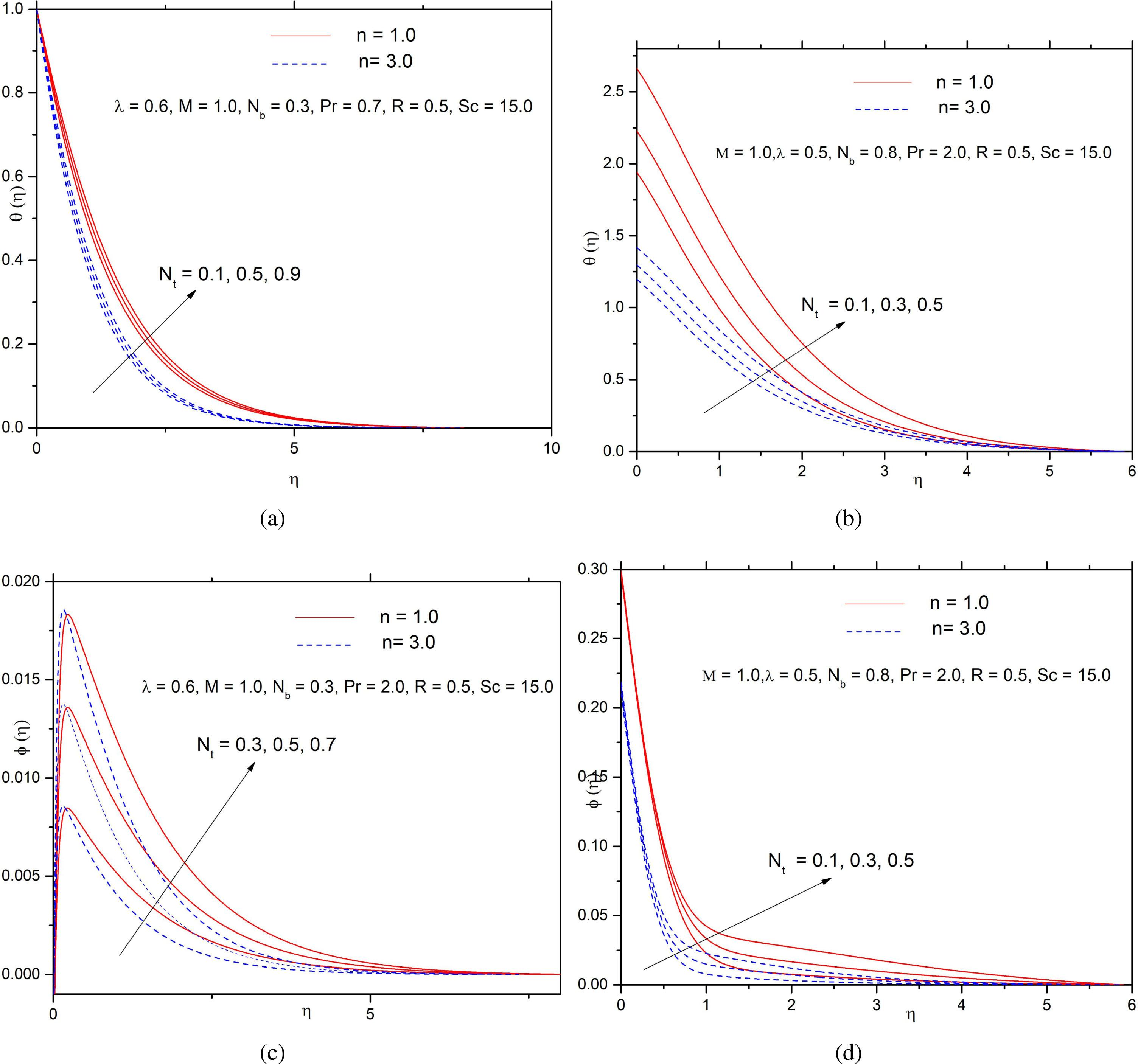Influences of thermophoresis parameter Nt on temperature θ (η) for (a) CWT and (b) PHF and on ϕ(η), nanoparticle volume fraction for (c) ZMF and (d) PMF.

The influences of radiation on temperature and nanoparticle volume fraction are portrayed in Figures 6(a–d). It is observed that the temperature [Figures 6(a,b)] and the nanoparticle volume fraction [Figures 6(c,d)] both increase for a rise in R. As Ke diminishes when the thermal radiation parameter R increases, which, in turn, causes to amplify the deviation of the heat flux due to radiation. The speed of heat transport due to radiation augments and consequently the fluid temperature increases. The width of the temperature boundary layer enlarges by a rise in heat transport to the liquid due to radiation.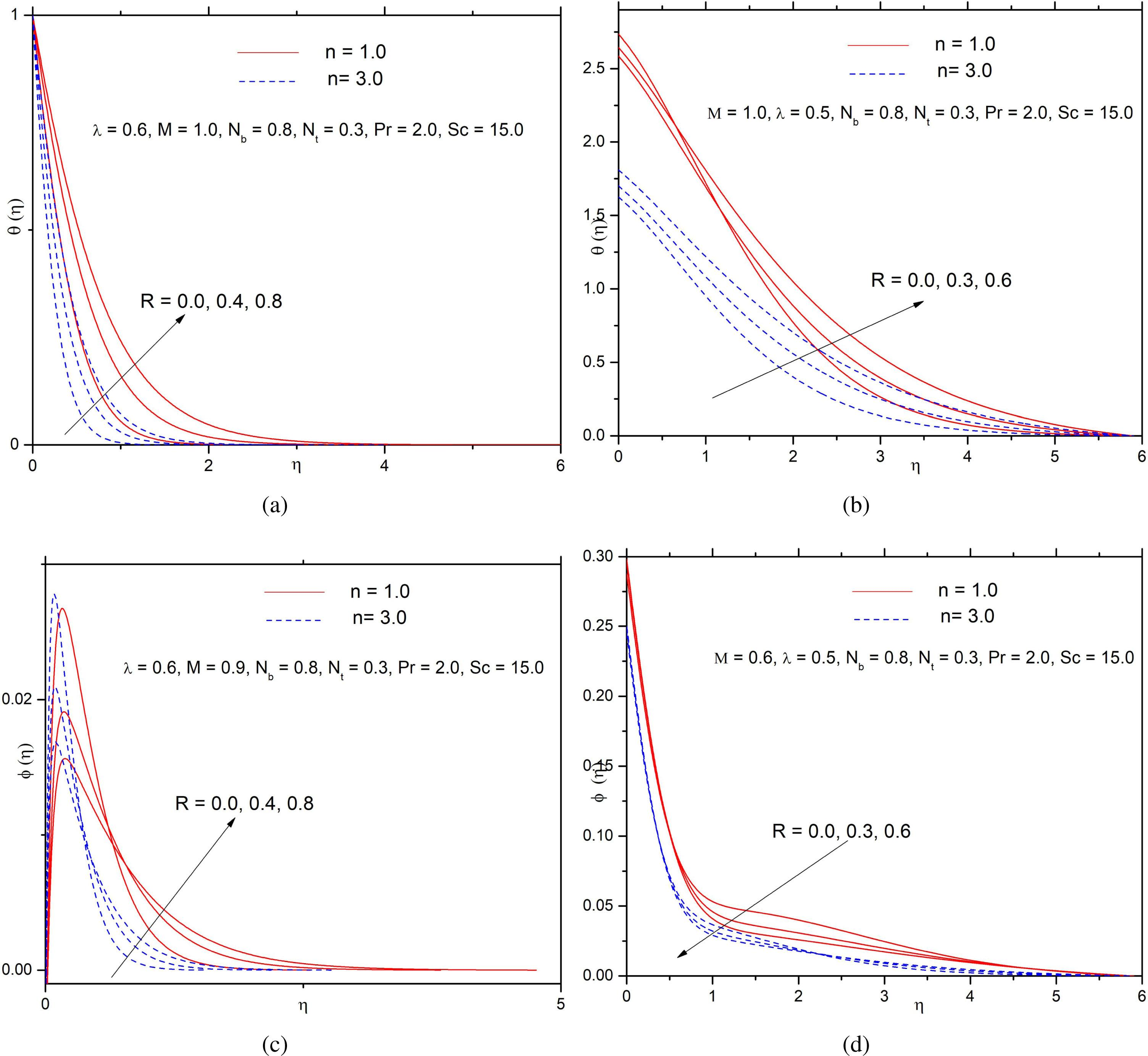Influences of R, radiation parameter on temperature θ (η) for (a) CWT and (b) PHF and on nanoparticle volume fraction ϕ(η) for (c) ZMF and (d) PMF.

The effects of the Prandtl number Pr on temperature and concentration fields of the nanofluid are presented in Figures 7(a–d). The thicknesses of both the boundary layers: thermal [Figures 7(a,b)] and solutal [Figures 7(c,d)] diminish for the higher values of Pr. Owing to an augmentation in Pr, the thermal diffusivity reduces, and it reduces the energy transferability.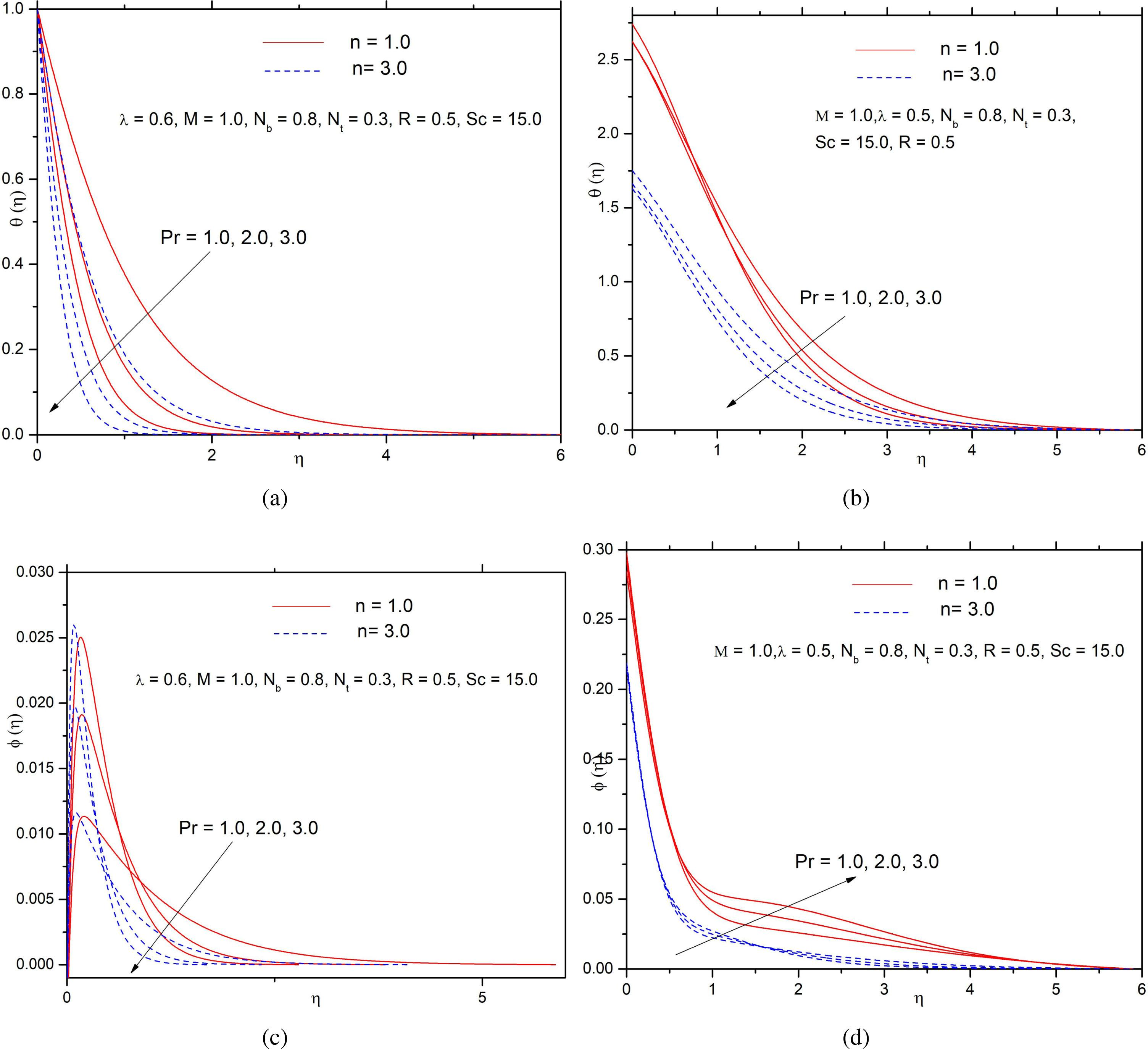Influences of Pr, Prandtl number on θ (η), temperature for (a) CWT and (b) PHF and on ϕ(η), nanoparticle volume fraction for (c) ZMF and (d) PMF.

Almost negligible effect in the temperature is seen due to changes in the Brownian motion parameter Nb. This agrees very well with the findings of Kuznetsov and Nield (2014) and Khan et al. (2015) for the CWT case. Due to a rise in the Brownian motion parameter Nb temperature significantly increases in the PHF case [Figure 8(a)]. On the other hand, the temperature drops down with a rise in the Schmidt number Sc for the PHF case [Figure 8(b)]. This effect is pronounced in the linearly stretching sheet [n = 1] case compared to non-linearly stretching sheet [n = 3] case. Reduction in the thickness of the solute boundary layer for higher values of Nb is experienced in both ZMF [Figure 9(a)] and PMF [Figure 9(b)] cases. It can be seen that due to a rise in the Schmidt number Sc, the width of the solute boundary layer reduces in both cases of ZMF [Figure 9(c)] and PMF [Figure 9(d)]. A significant decrease in concentration is noted for the non-linearly stretching sheet (n = 3) case compared to the linearly stretching sheet (n = 1) case. Schmidt number, Sc, is the relation of diffusivities related to momentum and mass. A higher value of Sc indicates the smaller value of coefficient DB of Brownian diffusion that opposes the nanoparticles to penetrate intensely into the liquid.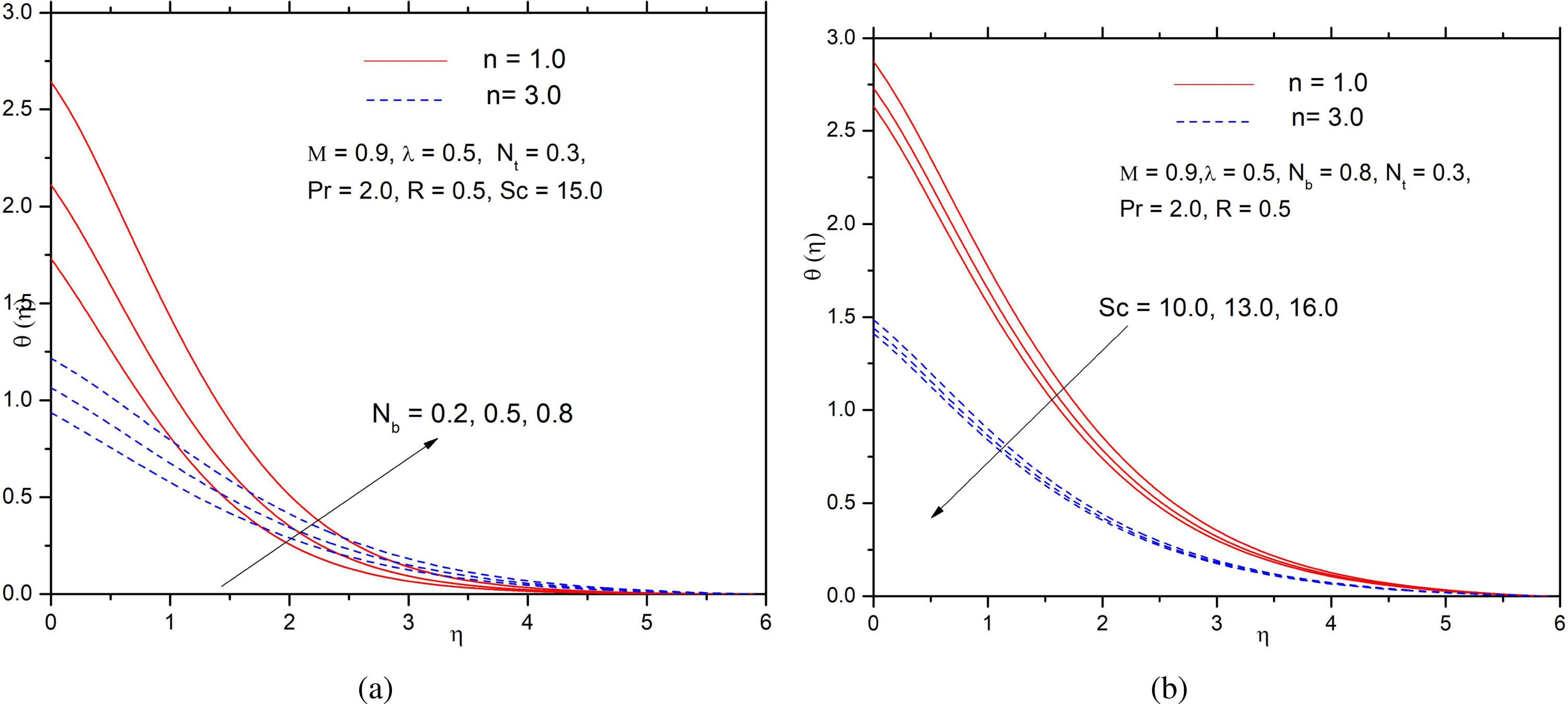Effects of (a) Nb, the parameter for Brownian motion and (b) Sc, the Schimidt number on temperature θ (η) for PHF.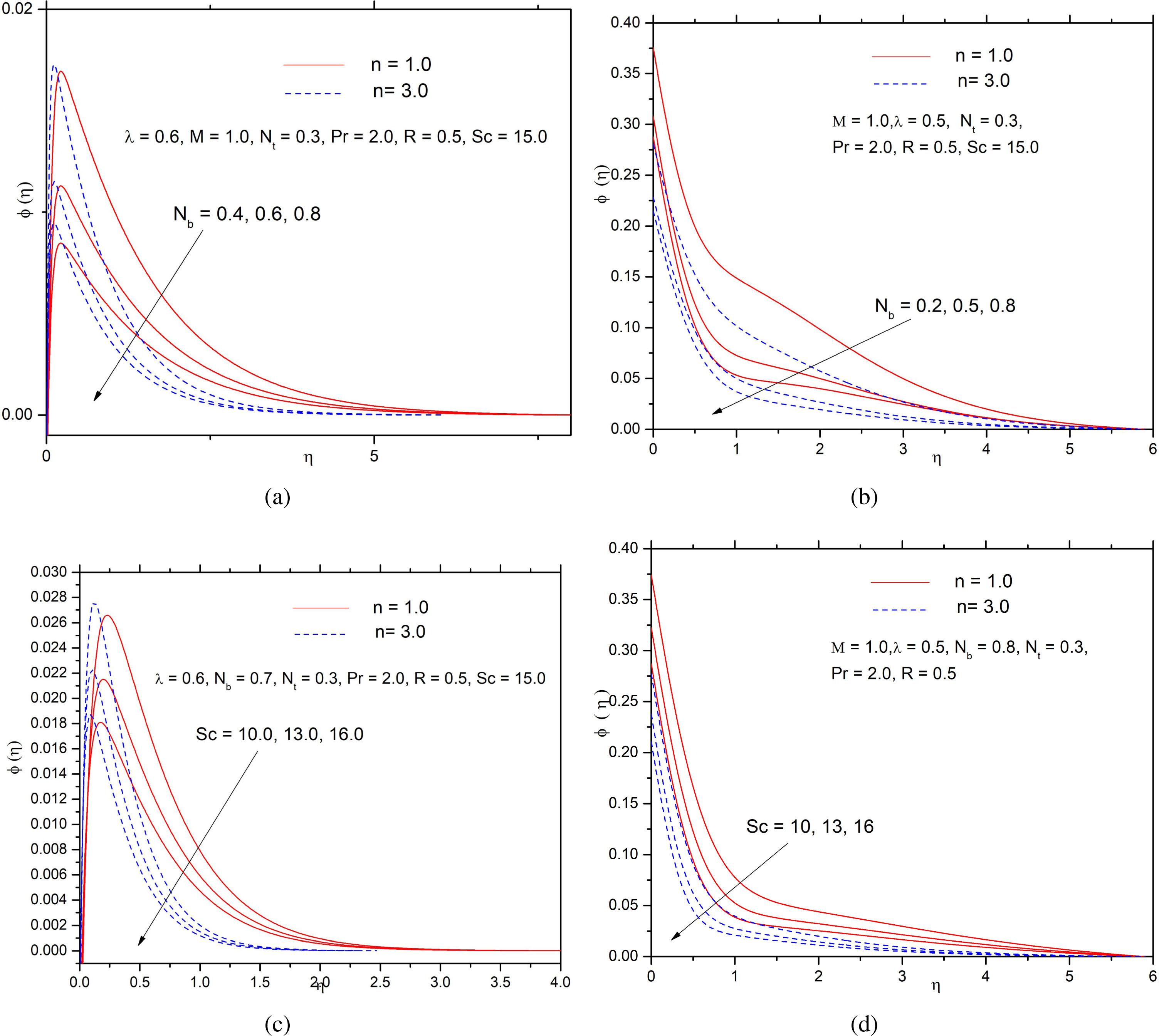Effects of Nb, the parameter for Brownian motion for (a) ZMF and (b) PMF and Schmidt number Sc for (c) ZMF and (d) PMF on nanoparticle volume fraction φ(η).

To provide a clear understanding of the flow field, the streamlines are presented for several values of n in Figures 10(a,b).

Figures 11(a,b) indicate that the coefficient of the skin friction (local) in the directions of x, y increases with increasing λ, M and n. From Figure 11(c), it is clear that the Nusselt number in the CWT case increases due to an increase in λ and n but decreases with the magnetic field parameter M. However, the local Sherwood number in the ZMF case increases with the magnetic parameter r but decreases with the stretching ratio parameter λ and with the power-law index n. These changes are depicted in Figure 11(d).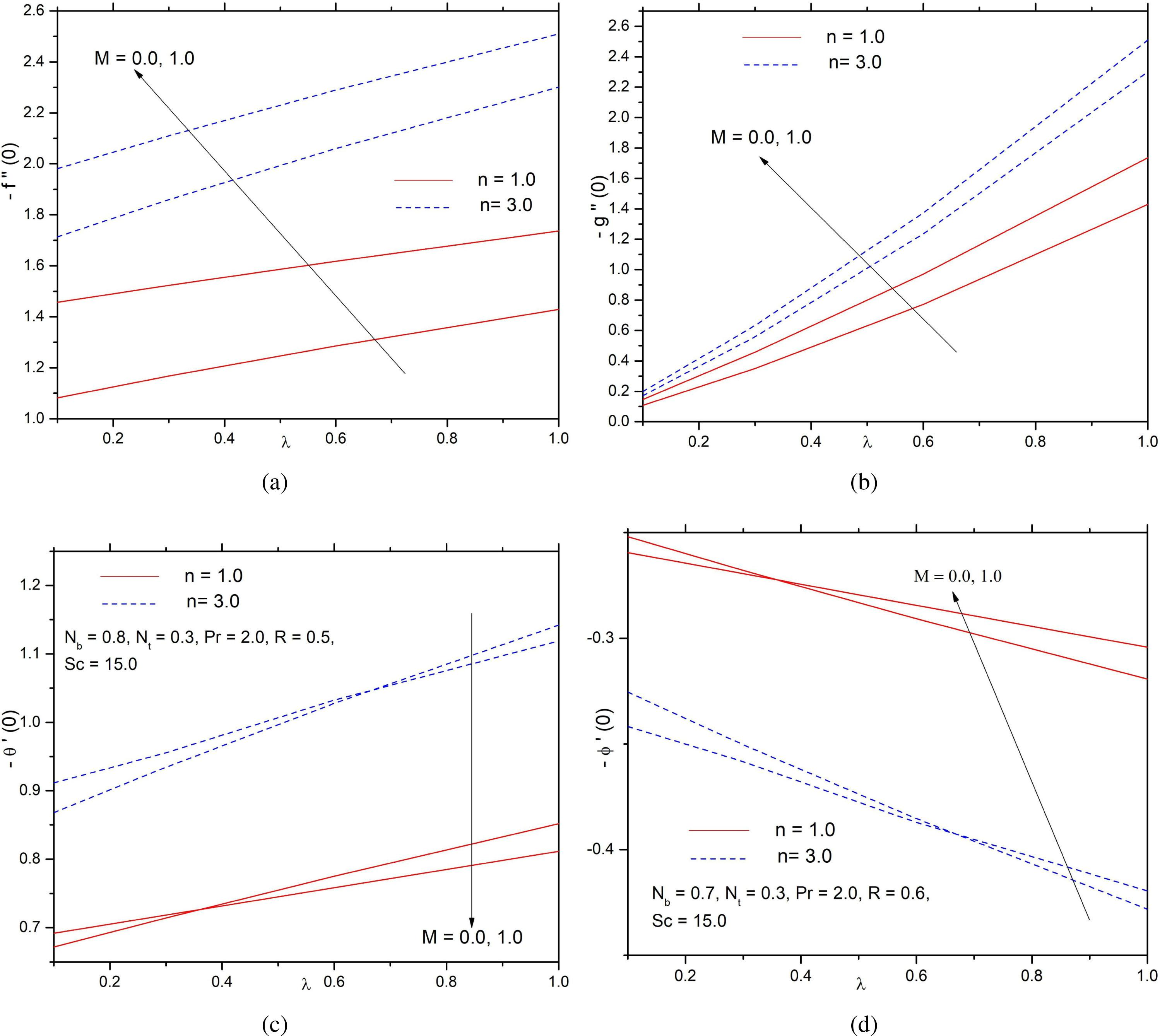Distributions of (a)− f″(0) (b)−g″(0) (c) −θ′ (0) [for CWT] (d) −ϕ′ (0) [for ZMF] with λ, stretching ratio parameter for diverse values of magnetic field parameter M.

The effects of Nb, Nt on the Nusselt number for the CWT case and local Sherwood number for ZMF case are portrayed in Figures 12(a,b), respectively. Both are found to increase with an increase in Nb.On the other hand, the Nusselt number [Figure 12(a)] decreases and Sherwood number [Figure 12(b)] increases with the increasing values of Nt.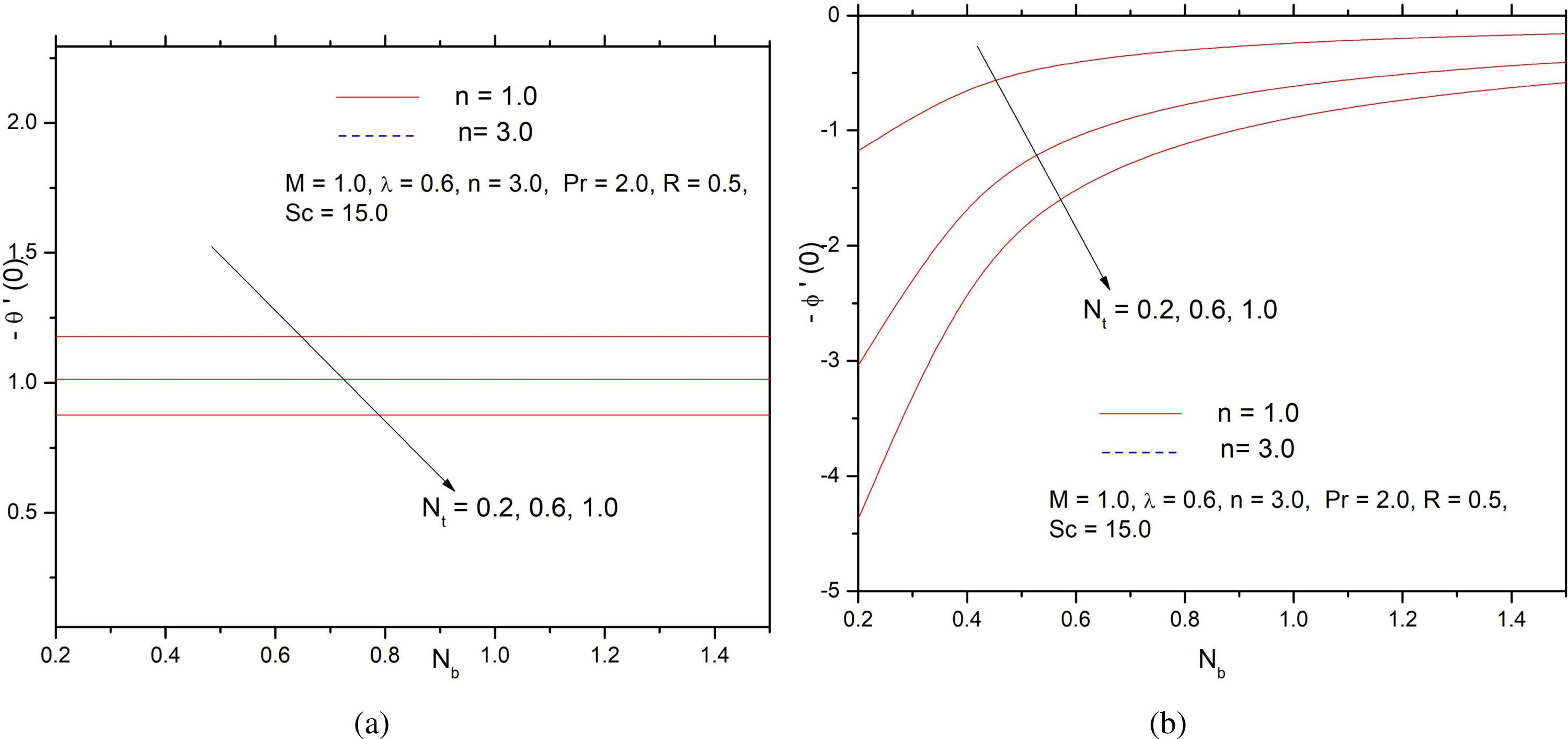Distributions of (a) −θ′ (0) for CWT (b) −ϕ′ (0) for ZMF with Nb, Brownian motion parameter for diverse values of Nt, thermophoresis parameter.

Table 3 shows that −θ′(0), −φ′(0) both decrease with the increasing values of the thermophoresis parameter. Also, −θ′(0) increases with the increasing Prandtl number but −φ′(0) decreases with Pr.

Values of −θ′(0) and −ϕ′(0) for different values of thermophoresis parameter Nt and Prandtl number Pr with n = 4.0, λ = 0.9, Nb = 0.7, Pr = 2.0, R = 0.5, Sc = 15.0

Nt Pr
1.0 1.5 2.0 2.5
θ′(0) ϕ′(0) θ′(0) ϕ′(0) θ′(0) ϕ′(0) θ′(0) ϕ′(0)
0.3 1.04746 −0.44891 1.29932 −0.55685 1.52625 −0.65416 1.72719 −0.744224
0.6 0.97822 −0.838474 1.18042 −1.01179 1.35314 −1.15983 1.4979 −1.28392
0.9 0.914269 −1.17549 1.07387 −1.08369 1.20209 −1.54554 1.30267 −1.67486
1.2 0.855295 −1.46622 0.978737 −1.67784 1.07114 −1.83624 1.13802 −1.9509
1.5 0.80098 −1.71639 0.894035 −1.91579 0.958127 −2.05313 1.00002 −2.14289
1.8 0.751003 −1.93115 0.818763 −2.10539 0.860844 −2.2136 0.88466 −2.27486
Concluding remarks

The current study provides solutions (numerical) for a steady MHD three-dimensional nanofluid flow past a bi-directional non-linearly stretching surface. Two types of conditions at the boundary for temperature and volume fraction for nanoparticles are considered. From the current investigation, the following observations are derived:

A rise in the magnetic strength causes a reduction in the resistance and the rate of heat transport.

Both the thermal and the concentration boundary layer thicknesses increase with an increase in the magnetic parameter in addition to the radiation parameter.

The volume fraction of the nanoparticles is a decreasing function of the Brownian motion parameter.

In the presence of thermophoresis, both temperature and volume fraction of the nanoparticles increase significantly in the boundary layer.

#### Comparisons for the values of f″(0) and g″(0) in the absence of magnetic field

N λ f″(0) g″(0)
Khan et al. (2015) Raju et al. (2016) Present study Khan et al. (2015) Raju et al. (2016) Present study
1 0 −1 −1 −1 0 0 0
0.5 −1.22474 −1.2247431 −1.2247429 −0.612372 −0.6123721 −0.6123720
1.0 −1.414214 −1.4142141 −1.4142140 −1.414214 −1.4142140 −1.4142140
3 0 −1.624356 −1.6243560 −1.6243560 0 0 0
0.5 −1.989422 −1.9894221 −1.9894220 −0.994711 −0.9947110 −0.9947110
1.0 −2.297186 −2.2971860 −2.2971860 −2.297186 −2.2971860 −2.2971860

#### Comparisons of the values of f″(0) and g″(0) in the absence of the magnetic field for different grid sizes

N f″(0) g″(0)
Grid size 0.01 Grid size 0.03 Grid size 0.01 Grid size 0.03
1 0 −1 −1 0 0
0.5 −1.2247429 −1.2247429 −0.6123720 −0.6123720
1.0 −1.4142140 −1.4142140 −1.4142140 −1.4142140
3 0 −1.6243560 −1.6243560 0 0
0.5 −1.9894220 −1.9894220 −0.9947110 −0.9947110
1.0 −2.2971860 −2.2971860 −2.2971860 −2.2971860

#### Values of −θ′(0) and −ϕ′(0) for different values of thermophoresis parameter Nt and Prandtl number Pr with n = 4.0, λ = 0.9, Nb = 0.7, Pr = 2.0, R = 0.5, Sc = 15.0

Nt Pr
1.0 1.5 2.0 2.5
θ′(0) ϕ′(0) θ′(0) ϕ′(0) θ′(0) ϕ′(0) θ′(0) ϕ′(0)
0.3 1.04746 −0.44891 1.29932 −0.55685 1.52625 −0.65416 1.72719 −0.744224
0.6 0.97822 −0.838474 1.18042 −1.01179 1.35314 −1.15983 1.4979 −1.28392
0.9 0.914269 −1.17549 1.07387 −1.08369 1.20209 −1.54554 1.30267 −1.67486
1.2 0.855295 −1.46622 0.978737 −1.67784 1.07114 −1.83624 1.13802 −1.9509
1.5 0.80098 −1.71639 0.894035 −1.91579 0.958127 −2.05313 1.00002 −2.14289
1.8 0.751003 −1.93115 0.818763 −2.10539 0.860844 −2.2136 0.88466 −2.27486

L. J. Crane, Flow past a stretching plate, Zeitschrift für Angewandte Mathematik und Physik ZAMP, 21(4) (1970) 645–647. CraneL. J. Flow past a stretching plate Zeitschrift für Angewandte Mathematik und Physik ZAMP 21 4 1970 645 647 10.1007/BF01587695 Search in Google Scholar

P. S. Gupta and A. S. Gupta, Heat and mass transfer on a stretching sheet with suction or blowing, Can J Chem Eng, 55 (1977) 744–746. GuptaP. S. GuptaA. S. Heat and mass transfer on a stretching sheet with suction or blowing Can J Chem Eng 55 1977 744 746 10.1002/cjce.5450550619 Search in Google Scholar

M.A. Hamad and I. Pop, Scaling transformations for boundary layer flow near the stagnation-point on a heated permeable stretching surface in a porous medium saturated with a nanofluid and heat generation/absorption effects, Trans Porous Medium, 87 (2010) 25–39. HamadM.A. PopI. Scaling transformations for boundary layer flow near the stagnation-point on a heated permeable stretching surface in a porous medium saturated with a nanofluid and heat generation/absorption effects Trans Porous Medium 87 2010 25 39 10.1007/s11242-010-9683-8 Search in Google Scholar

M. Turkyilmazoglu, The analytical solution of mixed convection heat transfer and fluid flow of a MHD viscoelastic fluid over a permeable stretching surface, Int J Mech Sci, 77 (2013) 263–268. TurkyilmazogluM. The analytical solution of mixed convection heat transfer and fluid flow of a MHD viscoelastic fluid over a permeable stretching surface Int J Mech Sci 77 2013 263 268 10.1016/j.ijmecsci.2013.10.011 Search in Google Scholar

D. Pal and G. Mandal, Mixed convection-radiation on stagnation-point flow of nanofluids over a stretching/shrinking sheet in a porous medium with heat generation and viscous dissipation, J Petrol Sci Eng, 126 (2015) 16–25. PalD. MandalG. Mixed convection-radiation on stagnation-point flow of nanofluids over a stretching/shrinking sheet in a porous medium with heat generation and viscous dissipation J Petrol Sci Eng 126 2015 16 25 10.1016/j.petrol.2014.12.006 Search in Google Scholar

M. Waqas, M. Farooq, M.I. Khan, A. Alsaedi, T. Hayat, T. Yasmeen, Magnetohydrodynamic (MHD) mixed convection flow of micropolar liquid due to nonlinear stretched sheet with convective condition, Int J Heat Mass Transfer 102 (2016) 766–772. WaqasM. FarooqM. KhanM.I. AlsaediA. HayatT. YasmeenT. Magnetohydrodynamic (MHD) mixed convection flow of micropolar liquid due to nonlinear stretched sheet with convective condition Int J Heat Mass Transfer 102 2016 766 772 10.1016/j.ijheatmasstransfer.2016.05.142 Search in Google Scholar

J. Buongiorno, Convective transport in nanofluids, ASME J Heat Transf, 128, (2006) 240–250. BuongiornoJ. Convective transport in nanofluids ASME J Heat Transf 128 2006 240 250 10.1115/1.2150834 Search in Google Scholar

H. Masuda, A. Ebata, K. Teramae and N. Hishinuma, Alteration of thermal conductivity and viscosity of liquid by dispersing ultra-fine particles (dispersion of c-Al2O3, SiO2 and TiO2 ultra-fine particles), NetsuBussei (in Japanese), 4 (1993) 227–233. MasudaH. EbataA. TeramaeK. HishinumaN. Alteration of thermal conductivity and viscosity of liquid by dispersing ultra-fine particles (dispersion of c-Al2O3, SiO2 and TiO2 ultra-fine particles) NetsuBussei (in Japanese) 4 1993 227 233 10.2963/jjtp.7.227 Search in Google Scholar

S.U.S. Choi and J.A. Eastman, Enhancing thermal conductivity of fluids with nanoparticles, The Proceedings of the 1995 ASME International Mechanical Engineering Congress and Exposition, San Francisco, USA, ASME, FED 231/MD, 66, (1995), 99–105. ChoiS.U.S. EastmanJ.A. Enhancing thermal conductivity of fluids with nanoparticles The Proceedings of the 1995 ASME International Mechanical Engineering Congress and Exposition, San Francisco, USA, ASME, FED 231/MD 66 1995 99 105 Search in Google Scholar

K. Das, Slip flow and convective heat transfer of nanofluids over a permeable stretching surface, Comput. Fluids, 64 (2012) 34–42. DasK. Slip flow and convective heat transfer of nanofluids over a permeable stretching surface Comput. Fluids 64 2012 34 42 10.1016/j.compfluid.2012.04.026 Search in Google Scholar

P. Rana and R. Bhargava, Flow and heat transfer of a nanofluid over a nonlinearly stretching sheet: A numerical study, Comm Nonlinear Sci Num Simul, 17 (2012) 212–226. RanaP. BhargavaR. Flow and heat transfer of a nanofluid over a nonlinearly stretching sheet: A numerical study Comm Nonlinear Sci Num Simul 17 2012 212 226 10.1016/j.cnsns.2011.05.009 Search in Google Scholar

S. Nadeem, C. Lee, Boundary layer flow of nanofluid over an exponentially stretching surface, Nanoscale Research Lett, 7(2012) 94. NadeemS. LeeC. Boundary layer flow of nanofluid over an exponentially stretching surface Nanoscale Research Lett 7 2012 94 10.1186/1556-276X-7-94330550222289390 Search in Google Scholar

M. Mustafa, T. Hayat and S. Obaidat, Boundary layer flow of a nanofluid over an exponentially stretching sheet with convective boundary conditions, Int J Numer Meth Heat Fluid Flow 23 (2013) 945–959. MustafaM. HayatT. ObaidatS. Boundary layer flow of a nanofluid over an exponentially stretching sheet with convective boundary conditions Int J Numer Meth Heat Fluid Flow 23 2013 945 959 10.1108/HFF-09-2011-0179 Search in Google Scholar

S. Nadeem, R. Ul Haq and Z. H. Khan, Numerical Solution of Non-Newtonian Nanofluid Flow over a Stretching Sheet, Appl Nanosci, 4 (2014), 625–631. NadeemS. Ul HaqR. KhanZ. H. Numerical Solution of Non-Newtonian Nanofluid Flow over a Stretching Sheet Appl Nanosci 4 2014 625 631 10.1007/s13204-013-0235-8 Search in Google Scholar

T. Hayat, A. Kiran, M. Imtiaz, A. Alsaedi, Hydromagnetic mixed convection flow of copper and silver water nanofluids due to a curved stretching sheet, Results in Physics, 6 (2016) 904–910. HayatT. KiranA. ImtiazM. AlsaediA. Hydromagnetic mixed convection flow of copper and silver water nanofluids due to a curved stretching sheet Results in Physics 6 2016 904 910 10.1016/j.rinp.2016.10.023 Search in Google Scholar

Md. S. Khan, Md. M. Alam and M. Ferdows, Effects of magnetic field on radiative flow of a nanofluid past a stretching sheet, Procedia Engng, 56 (2013) 316 – 322. KhanMd. S. AlamMd. M. FerdowsM. Effects of magnetic field on radiative flow of a nanofluid past a stretching sheet Procedia Engng 56 2013 316 322 10.1016/j.proeng.2013.03.125 Search in Google Scholar

M. Sheikholeslami, M.G. Bandpy, R. Ellahi, M. Hassan and S. Soleimani, Effects of MHD on Cu—water nanofluid flow and heat transfer by means of CVFEM, J Magn Magn Mater, 349 (2014) 188–200. SheikholeslamiM. BandpyM.G. EllahiR. HassanM. SoleimaniS. Effects of MHD on Cu—water nanofluid flow and heat transfer by means of CVFEM J Magn Magn Mater 349 2014 188 200 10.1016/j.jmmm.2013.08.040 Search in Google Scholar

M. Sheikholeslami, D. D. Ganji, M. M. Rashidi, Magnetic field effect on unsteady nanofluid flow and heat transfer using Buongiorno model, J Mag Mag Mater, 416 (2016) 164–173. SheikholeslamiM. GanjiD. D. RashidiM. M. Magnetic field effect on unsteady nanofluid flow and heat transfer using Buongiorno model J Mag Mag Mater 416 2016 164 173 10.1016/j.jmmm.2016.05.026 Search in Google Scholar

Yuan Ma, R. Mohebbi, M. M. Rashidi, Z. Yang, MHD convective heat transfer of Ag-MgO/water hybrid nanofluid in a channel with active heaters and coolers, Int Journal of Heat and Mass Transfer, 137 (2019) 714–726. MaYuan MohebbiR. RashidiM. M. YangZ. MHD convective heat transfer of Ag-MgO/water hybrid nanofluid in a channel with active heaters and coolers Int Journal of Heat and Mass Transfer 137 2019 714 726 10.1016/j.ijheatmasstransfer.2019.03.169 Search in Google Scholar

Yuan Ma, R. Mohebbi, M. M. Rashidi, O Manca, Z. Yang, Numerical investigation of MHD effects on nanofluid heat transferin a baffled U-shaped enclosure using lattice Boltzmann method, Journal of thermal analysis and Calorimetry, 135 (2019) 3197–3213. MaYuan MohebbiR. RashidiM. M. MancaO YangZ. Numerical investigation of MHD effects on nanofluid heat transferin a baffled U-shaped enclosure using lattice Boltzmann method Journal of thermal analysis and Calorimetry 135 2019 3197 3213 10.1007/s10973-018-7518-y Search in Google Scholar

M. M. Bhatti, S. R. Mishra, T. Abbas, M. M. Rashidi, A mathematical model of MHD nanofluid flow having gyrotactic microorganisms with thermal radiation and chemical reaction effects, Neural Computing and Applications, 30(4) (2018) 1237–1249. BhattiM. M. MishraS. R. AbbasT. RashidiM. M. A mathematical model of MHD nanofluid flow having gyrotactic microorganisms with thermal radiation and chemical reaction effects Neural Computing and Applications 30 4 2018 1237 1249 10.1007/s00521-016-2768-8 Search in Google Scholar

K. Das, P. R. Duari, P. K. Kundu, Nanofluid flow over an unsteady stretching surface in presence of thermal radiation, Alexandria Engng J, 53 (2014) 737–745. DasK. DuariP. R. KunduP. K. Nanofluid flow over an unsteady stretching surface in presence of thermal radiation Alexandria Engng J 53 2014 737 745 10.1016/j.aej.2014.05.002 Search in Google Scholar

T. Hayat, M. Waqas, S. A. Shehzad, A. Alsaedi, A model of solar radiation and Joule heating in magnetohydrodynamic (MHD) convective flow of thixotropic nanofluid, J Mol Liq, 15 (2016) 704–710. HayatT. WaqasM. ShehzadS. A. AlsaediA. A model of solar radiation and Joule heating in magnetohydrodynamic (MHD) convective flow of thixotropic nanofluid J Mol Liq 15 2016 704 710 10.1016/j.molliq.2016.01.005 Search in Google Scholar

T. Hayat, A. Shafiq, A. Alsaedi, Hydromagnetic boundary layer flow of Williamson fluid in the presence of thermal radiation and Ohmic dissipation, Alexandria Engng J, 55 (2016) 2229–2240. HayatT. ShafiqA. AlsaediA. Hydromagnetic boundary layer flow of Williamson fluid in the presence of thermal radiation and Ohmic dissipation Alexandria Engng J 55 2016 2229 2240 10.1016/j.aej.2016.06.004 Search in Google Scholar

C.Y. Wang, The three-dimensional flow due to a stretching flat surface, Phys Fluids, 27 (8) (1984) 1915. WangC.Y. The three-dimensional flow due to a stretching flat surface Phys Fluids 27 8 1984 1915 10.1063/1.864868 Search in Google Scholar

P.D. Ariel, The three-dimensional flow past a stretching sheet and the homotopy perturbation method, Comput Math Appl, 54 (7–8) (2007) 920–925. ArielP.D. The three-dimensional flow past a stretching sheet and the homotopy perturbation method Comput Math Appl 54 7–8 2007 920 925 10.1016/j.camwa.2006.12.066 Search in Google Scholar

I.C. Liu and H. I. Andersson, Heat transfer over a bidirectional stretching sheet with variable thermal conditions, Int J Heat Mass Transf, 51 (2008) 4018–4024. LiuI.C. AnderssonH. I. Heat transfer over a bidirectional stretching sheet with variable thermal conditions Int J Heat Mass Transf 51 2008 4018 4024 10.1016/j.ijheatmasstransfer.2007.10.041 Search in Google Scholar

K. Ahmad and R. Nazar, Magnetohydrodynamic three dimensional flow and heat transfer over a stretching surface in a viscoelastic fluid, J of science and technology, 3 (1) (2011). AhmadK. NazarR. Magnetohydrodynamic three dimensional flow and heat transfer over a stretching surface in a viscoelastic fluid J of science and technology 3 1 2011 Search in Google Scholar

I.C. Liu, H.H. Wang and Y.F. Peng, Flow and heat transfer for three dimensional flow over an exponentially stretching surface, Chem Eng Comm, 200 (2013) 253–268. LiuI.C. WangH.H. PengY.F. Flow and heat transfer for three dimensional flow over an exponentially stretching surface Chem Eng Comm 200 2013 253 268 10.1080/00986445.2012.703148 Search in Google Scholar

T. Hayat, S.A. Shehzad, A. Alsaedi and M.S. Alhothuali, Three dimensional of Oldroyd-B fluid over surface with convective boundary conditions, Appl Math Mech, 34 (4) (2013) 489–500. HayatT. ShehzadS.A. AlsaediA. AlhothualiM.S. Three dimensional of Oldroyd-B fluid over surface with convective boundary conditions Appl Math Mech 34 4 2013 489 500 10.1007/s10483-013-1685-9 Search in Google Scholar

S. Nadeem, Rizwan Ul Haq, Noreen Sher Akbar, Z.H. Khan, MHD three-dimensional Casson fluid flow past a porous linearly stretching sheet, Alexandria Engng J, 52 (2013) 577–582. NadeemS. Ul HaqRizwan AkbarNoreen Sher KhanZ.H. MHD three-dimensional Casson fluid flow past a porous linearly stretching sheet Alexandria Engng J 52 2013 577 582 10.1016/j.aej.2013.08.005 Search in Google Scholar

J. A. Khan, M. Mustafa, T. Hayat, A. Alsaedi, On Three-Dimensional Flow and Heat Transfer over a Non-Linearly Stretching Sheet: Analytical and Numerical Solutions, PLOS ONE, 9 (9) (September 2014), e107287. KhanJ. A. MustafaM. HayatT. AlsaediA. On Three-Dimensional Flow and Heat Transfer over a Non-Linearly Stretching Sheet: Analytical and Numerical Solutions PLOS ONE 9 9 September 2014 e107287 10.1371/journal.pone.0107287415786425198696 Search in Google Scholar

J.A. Khan, M. Mustafa, T. Hayat and A. Alsaedi, Three dimensional flow of nanofluid over a nonlinearly stretching sheet: an application to solar energy, Int J Heat Mass Trans, 86 (2015) 158–164. KhanJ.A. MustafaM. HayatT. AlsaediA. Three dimensional flow of nanofluid over a nonlinearly stretching sheet: an application to solar energy Int J Heat Mass Trans 86 2015 158 164 10.1016/j.ijheatmasstransfer.2015.02.078 Search in Google Scholar

J. A. Khan, M. Mustafa, T. Hayat, M. Sheikholeslami, A. Alsaedi, Three-Dimensional Flow of Nanofluid Induced by an Exponentially Stretching Sheet: An Application to Solar Energy, PLOS ONE, DOI:10.1371/journal.pone.0116603 March 18, 2015 KhanJ. A. MustafaM. HayatT. SheikholeslamiM. AlsaediA. Three-Dimensional Flow of Nanofluid Induced by an Exponentially Stretching Sheet: An Application to Solar Energy PLOS ONE 10.1371/journal.pone.0116603 March 18 2015 436498725785857

C.S.K. Raju, N. Sandeep, M. Jayachandra Babu and V. Sugunamma, Dual solutions for three-dimensional MHD flow of a nanofluid over a nonlinearly permeable stretching sheet, Alexandria Engng J, 55 (2016) 151–162. RajuC.S.K. SandeepN. Jayachandra BabuM. SugunammaV. Dual solutions for three-dimensional MHD flow of a nanofluid over a nonlinearly permeable stretching sheet Alexandria Engng J 55 2016 151 162 10.1016/j.aej.2015.12.017 Search in Google Scholar

S. A. Shehzad, Z. Abdullah, A. Alsaedi, F. M. Abbasi, T. Hayat, Thermally radiative three-dimensional flow of Jeffrey nanofluid with internal heat generation and magnetic field, J Magnet Magn Mater, 397 (2016) 108–114. ShehzadS. A. AbdullahZ. AlsaediA. AbbasiF. M. HayatT. Thermally radiative three-dimensional flow of Jeffrey nanofluid with internal heat generation and magnetic field J Magnet Magn Mater 397 2016 108 114 10.1016/j.jmmm.2015.07.057 Search in Google Scholar

T. Hayat, S. Qayyum, S. A. Shehzad, A. Alsaedi, Simultaneous effects of heat generation/absorption and thermal radiation in magnetohydrodynamics (MHD) flow of Maxwell nanofluid towards a stretched surface, Results Phys, 2017, http://dx.doi.org/10.1016/j.rinp.2016.12.009 HayatT. QayyumS. ShehzadS. A. AlsaediA. Simultaneous effects of heat generation/absorption and thermal radiation in magnetohydrodynamics (MHD) flow of Maxwell nanofluid towards a stretched surface Results Phys 2017 http://dx.doi.org/10.1016/j.rinp.2016.12.009 10.1016/j.rinp.2016.12.009 Search in Google Scholar

S. Mansur, A. Ishak, The flow and heat transfer of a nanofluid past a stretching/shrinking sheet with convective boundary condition, Abstract Appl Anal, 350647 (2013). MansurS. IshakA. The flow and heat transfer of a nanofluid past a stretching/shrinking sheet with convective boundary condition Abstract Appl Anal 350647 2013 10.1155/2013/350647 Search in Google Scholar

D.A. Nield, A.V. Kuznetsov, The one set of convection in a horizontal layer of finite depth, a revised model, Int J Heat Mass Transfer, 77 (2014) 915–918. NieldD.A. KuznetsovA.V. The one set of convection in a horizontal layer of finite depth, a revised model Int J Heat Mass Transfer 77 2014 915 918 10.1016/j.ijheatmasstransfer.2014.06.020 Search in Google Scholar

A.V. Kuznetsov, D.A. Nield, Natural convective boundary layer flow of nanofluid past a vertical plate: a revised model, Int J Therm Sci, 77 (2014) 126–129. KuznetsovA.V. NieldD.A. Natural convective boundary layer flow of nanofluid past a vertical plate: a revised model Int J Therm Sci 77 2014 126 129 10.1016/j.ijthermalsci.2013.10.007 Search in Google Scholar

M. Mustafa, J.A. Khan, T. Hayat, A. Alsaedi, Analytical and numerical solutions for axisymmetric flow of nanofluid due to non-linearly stretching sheet, Int J Non-Linear Mech, 71 (2015) 21–29. MustafaM. KhanJ.A. HayatT. AlsaediA. Analytical and numerical solutions for axisymmetric flow of nanofluid due to non-linearly stretching sheet Int J Non-Linear Mech 71 2015 21 29 10.1016/j.ijnonlinmec.2015.01.005 Search in Google Scholar

B. Mahanthesh, B.J. Gireesha, R. S. R. Gorla, Nonlinear radiative heat transfer in MHD three-dimensional flow of water based nanofluid over a non-linearly stretching sheet with convective boundary condition, J Nigerian Math Soc, 35 (2016) 178–198. MahantheshB. GireeshaB.J. GorlaR. S. R. Nonlinear radiative heat transfer in MHD three-dimensional flow of water based nanofluid over a non-linearly stretching sheet with convective boundary condition J Nigerian Math Soc 35 2016 178 198 10.1016/j.jnnms.2016.02.003 Search in Google Scholar

• #### Higher Education Agglomeration Promoting Innovation and Entrepreneurship Based on Spatial Dubin Model

Recommended articles from Trend MD